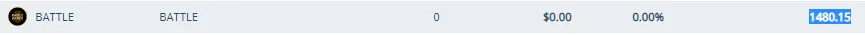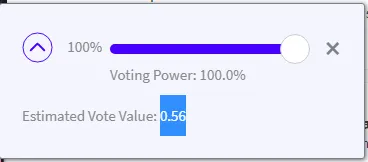This content got low rating by people.

Tokens power day 1 11/09/2021 part 1

The purpose of this account will be to store Hives as well as tokens (tokens in power)

day 11 / 365

Objectif :

Alive + 7.369
total = 150.695
Objectif = 1 000
vote value = 0.082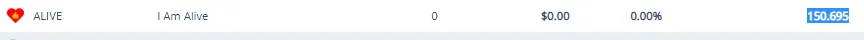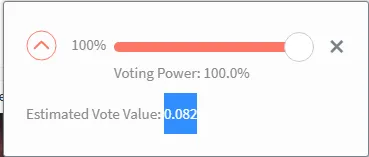Pob + 2.00078457
total = 2.9086325
Objectif = 1 000
vote value = 0.00061513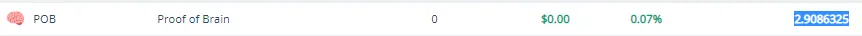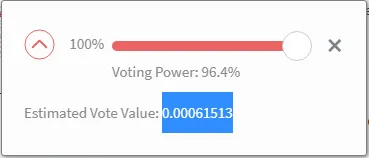archon + 0.55533259
total = 0.60074022
Objectif = 1 000
vote value = ???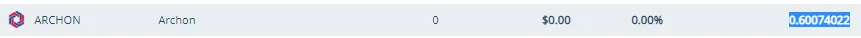lassecash + 0.02350046
total = 0.77350231
Objectif = 1 000
vote value = 0.00000051cine + 0.0010583
total = 2.82062818
Objectif = 1 000
vote value = 0.00181138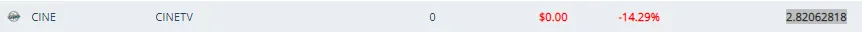Lern + 0.18858609
total = 128.650678
Objectif = 1 000
vote value = 0.61761763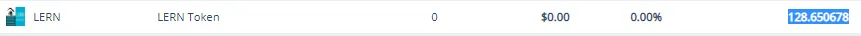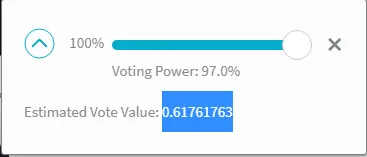BHT + 281.68626
total = 314.9577
Objectif = 1 000
vote value = 0.57264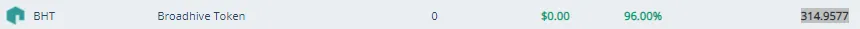BHT + 10.28
total = 1480.15
Objectif = 2 000
vote value = 0.56